### Home > MC2 > Chapter 10 > Lesson 10.1.2 > Problem10-21

10-21.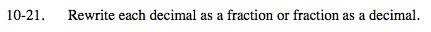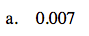Notice the place value of the decimal point.

What can you divide 7 by to get 0.007?

When you divide any number by 10, 100, 1000, etc. the decimal point moves to the left according to the number of zeros in the divisor.

$\frac{7}{1000}$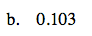Use the same method as in (a).

$\frac{103}{1000}$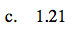Notice that 1.21 has a decimal point two decimal places to the left. Two decimal places refers to the hundredths place.

$\frac{121}{100}$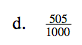You can conclude from (a) and (c) that dividing by a number consistingof multiple zeros moves the decimal left a number of times equal to the amount of zeros.

0.505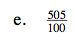Notice that the number 100 has two zeros.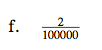How many zeros does the denominator have? How many times and in which direction should the decimal point move?